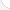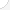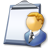Biomedical engineers Description: Apply knowledge of engineering, biology, and biomechanical principles to the design, development, and evaluation of biological and health systems and products, such as artificial organs, prostheses, instrumentation, medical information systems, and health management and care delivery systems.Source: Bureau of Labor Statistics, U.S. Department of Labor, Occupational Outlook Handbook, 2008-09 Edition at http://www.bls.gov/OCO/Complete Job Profile: http://www.bls.gov/oco/ocos027.htmSalary: \$50,001 or more per yearComments:

There are 36 math topics Biomedical engineers need to know.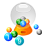Basic Math / Algebra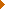FractionsDecimalsRatio and ProportionPercentCustomary MeasurementMetric MeasurementMeasurement ConversionBasic ProbabilityBasic StatisticsStatistical GraphingPowers and RootsOther Number BasesNegative NumbersScientific NotationBasic Problem Solving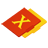First-Year AlgebraUsing FormulasLinear EquationsCoordinate Graphing 2DAlgebraic Representation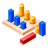GeometryGeometric MeanAreaVolume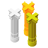Second-Year Algebra / TrigonometryVariationLogarithmsSequences and SeriesMatricesAdvanced ProbabilityAdvanced Statistics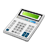Other TopicsBasic Calculator UseScientific Calculator UseComputer UseGroup Problem SolvingMental MathInductive/Deductive ReasoningMath CommunicationsMathematical Modeling